# 醉丶春风的Blog

## 用php实现一个跳表

### 什么是跳表

#### 理解跳表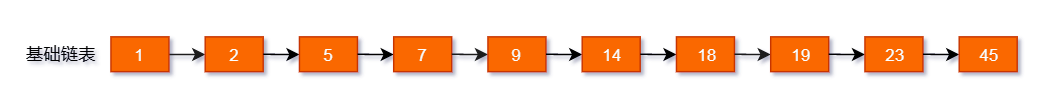#### 跳表模型图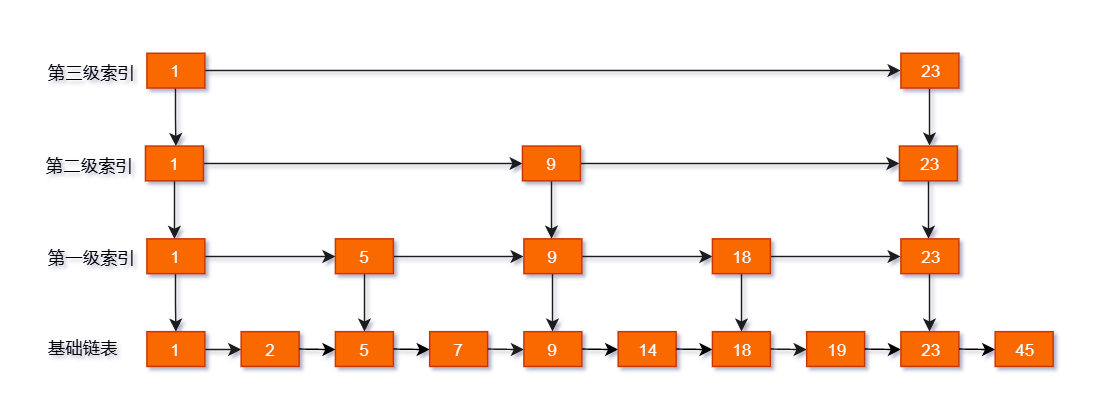#### 查询操作#### 插入操作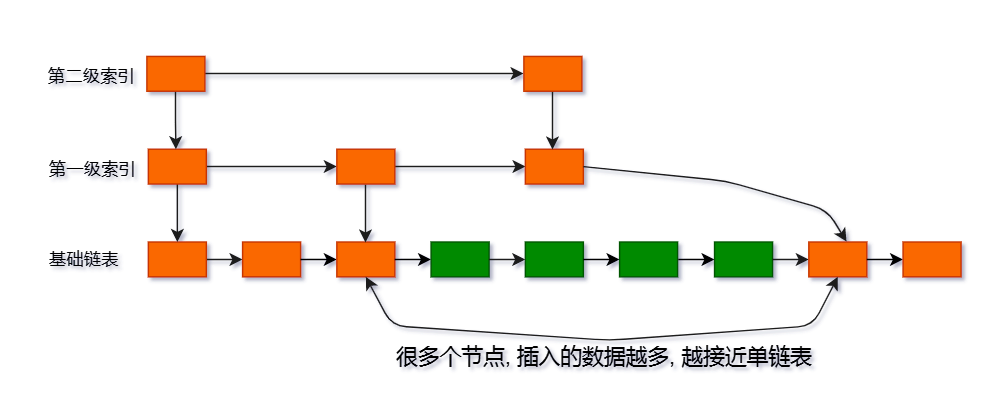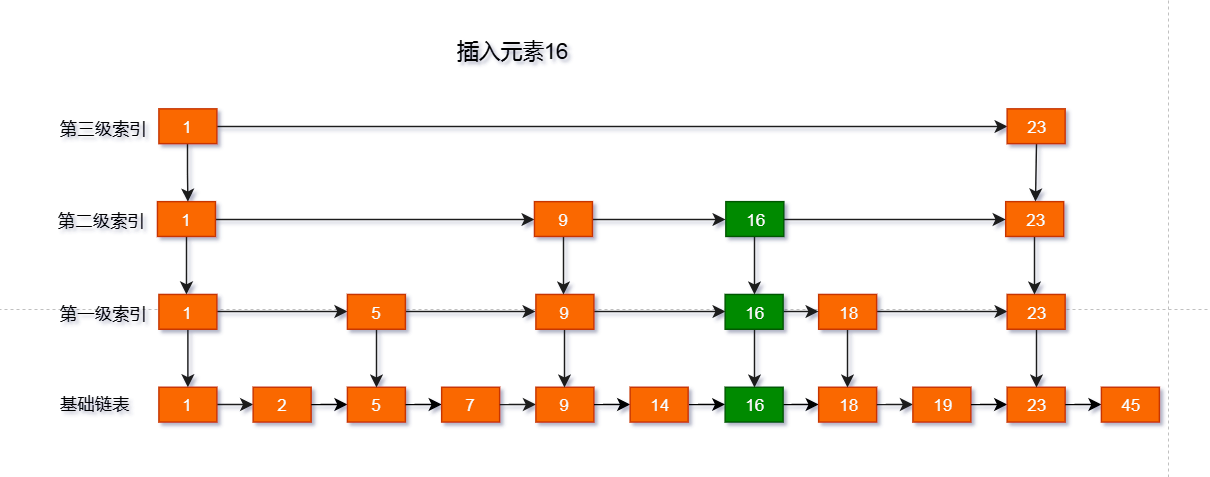#### 删除操作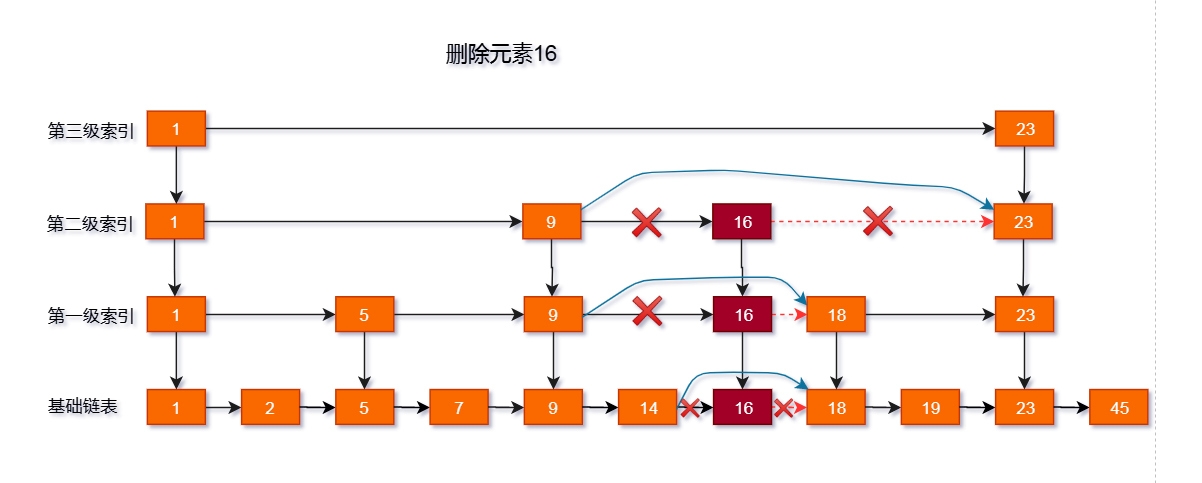### php代码实现

#### Node类

``````/**
* Class Node
* 跳表节点类
*/
class Node
{
/**
* @var int 节点数据
*/
public \$data;

/**
* @var Node[]
*/
public \$forward = [];

/**
* 代表该节点最多会出现在 \$maxLevel-1 层索引中
* @var int
*/
public \$maxLevel = 1;

public function __construct(\$data, \$forward = [], \$maxLevel = 1)
{
\$this->data = \$data;
\$this->forward = \$forward;
\$this->maxLevel = \$maxLevel;
}
}
``````

#### 跳表类

``````/**
* 跳表类
* Class SkipList
*/
class SkipList
{
/**
* @var Node 存储跳表入口, 每一级索引层的入口和数据层的入口
*/

/**
* @var int 当前跳表已经随机的最大层级
*/
public \$levelCount = 1;

/**
* 最大层级, 2^16=65536, 2^32=42亿+, 当16不够用时可选择更改
*/
const MAX_LEVEL = 16;

/**
* SkipList constructor.
* 初始化跳表的头结点, 定义 从 0 到 MAX_LEVEL-1 每一个元素的 下一个节点初始为null
*
* \$skipList = new SkipList();
* array(
'data' => -1,
'maxLevel' => MAX_LEVEL,
'forward' => array (
0 => NULL,
1 => NULL,
...,
MAX_LEVEL-1 => NULL,
),
)
*
*/
public function __construct()
{
\$node = new Node(-1, array_fill(0, self::MAX_LEVEL, null), self::MAX_LEVEL);
}

/**
* 插入数据
* 不管怎么样传数据, 插入后都会变成有序的
*
* 跳表示例:
* 如下跳表, \$levelCount应该等于3
* 1---------->9  索引层 第2级
* 1---->5---->9  索引层 第1级
* 1->3->5->7->9  数据层 第0级
*
* @param \$data
* @return \$this
*/
public function insert(\$data)
{
/**
* 计算本次插入的数据, 会更新到几级跳表索引, 最低为1, 因为跳表是从0开始的, 故计算时此level需要减1
* 数据层是第0层, level = 1 意味着只会写入到数据层, 不会创建跳表索引
*/
\$level = \$this->randomLevel();
// 等待被插入的数据, 它会出现在 从0 到 \$level - 1 层中
\$newNode = new Node(\$data, array_fill(0, \$level, null), \$level);

// 将跳表入口赋予\$p, 相当于指针
/**
* 等待被更新的数据
* 存储的是查询出新数据存放的位置的前一个元素, 将新数据的下一个节点指向下一个元素, 将前一个元素的下一个节点指向新数据
* 即可完成从数据层到索引层的插入
*/
\$update = [];
// 向下查找, 每一次循环都将下移一次索引层, 直到最后一次查询到数据层结束
for (\$i = \$level - 1; \$i >= 0; \$i--) {
// 向右查找, 横向查找索引层和数据层, 查找到第一个小于新数据的元素
while (\$p->forward[\$i] !== null && \$p->forward[\$i]->data < \$data) {
\$p = \$p->forward[\$i];
}
// 将第一个小于新数据的元素保存下来
\$update[\$i] = \$p;
}

/**
* 更新第一个小于新数据元素指针, 插入新元素
*/
for (\$i = 0; \$i < \$level; \$i++) {
\$newNode->forward[\$i] = \$update[\$i]->forward[\$i];
\$update[\$i]->forward[\$i] = \$newNode;
}

// 如果当前随机的level 大于当前跳表的level, 更新跳表的level
if (\$level > \$this->levelCount) {
\$this->levelCount = \$level;
}

return \$this;
}

/**
* 查找数据
* @param \$data
* @see insert() 参见该方法的查找说明
* @return bool|Node|mixed
*/
public function find(\$data)
{
for (\$i = \$this->levelCount - 1; \$i >= 0; \$i --) {
while (\$p->forward[\$i] !== null && \$p->forward[\$i]->data < \$data) {
\$p = \$p->forward[\$i];
}
}
if (\$p->forward !== null && \$p->forward->data == \$data) {
return \$p->forward;
}

return false;
}

/**
* 删除元素
* @param \$data
* @return bool
*/
public function delete(\$data)
{
// 跳表入口
/**
* 记录该元素所在层级的前一个元素, 存储的元素并不一定指向要删除的元素
*/
\$update = [];
for (\$i = \$this->levelCount - 1; \$i >= 0; \$i --) {
while (\$p->forward[\$i] !== null && \$p->forward[\$i]->data < \$data) {
\$p = \$p->forward[\$i];
}
\$update[\$i] = \$p;
}

/**
* 如果没有找到该元素
*/
if (\$p->forward === null || \$p->forward->data != \$data) {
return false;
}

/**
* 该元素最多会出现在它对应的maxLevel-1层中, 所以从这一层往下查找
* \$update中存储的都是 第一个小于 \$data的指针, 因此, 下一个元素不一定是\$data, 所以需要判断一下
*/
\$pLevel = \$p->forward->maxLevel;
for (\$i = \$pLevel - 1; \$i >= 0; \$i--) {
if (\$update[\$i]->forward[\$i] !== null && \$update[\$i]->forward[\$i]->data == \$data) {
\$update[\$i]->forward[\$i] = \$update[\$i]->forward[\$i]->forward[\$i];
}

}
/**
* 当被删除的元素处于最大层级时, 才检查删除该元素后是否需要减少层级
*/
if (\$this->levelCount == \$pLevel) {
// 当前层级的没有其他元素了, 说明该层级已空, 需要将当前最大层级减1
while (\$this->levelCount > 1 && \$this->head->forward[\$this->levelCount-1] === null) {
\$this->levelCount --;
}
}

return true;
}

/**
* 创建随机跳表层级
* \$p = 0.5 : 概率趋向于每2个元素提取一级索引
* \$p = 0.33 : 概率趋向于每3个元素提取一级索引
* \$p = 0.25 : 概率趋向于每4个元素提取一级索引
* ...
* `0xFFFF` = 65536, 参考redis zslRandomLevel函数
* @return int
*/
public function randomLevel()
{
\$level = 1;
\$p = 0.33;
while ((rand() & 0xFFFF) < \$p * 0xFFFF) {
\$level += 1;
}

return (\$level < self::MAX_LEVEL) ? \$level : self::MAX_LEVEL;
}

/**
* 打印跳表元素
*/
public function printAll()
{
echo '<hr>', PHP_EOL;

for (\$i = 0; \$i < \$this->levelCount; \$i++) {
echo sprintf('第 %d 级元素为: ', \$i);
while (\$p !== null) {
echo \$p->data, '->';

\$p = \$p->forward[\$i];
}

echo '<br>', PHP_EOL;
}
}
}
``````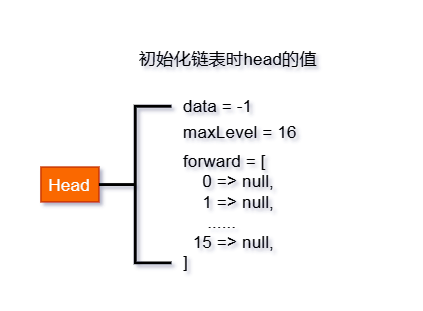#### 测试使用

``````\$skipList = new SkipList();
\$skipList->insert(2);
\$skipList->insert(5);
\$skipList->insert(7);
\$skipList->insert(9);
\$skipList->insert(20);
\$skipList->printAll();

\$skipList->delete(7);
\$skipList->delete(7);
\$skipList->delete(20);
\$skipList->printAll();
//var_dump(\$skipList->find(5));
var_dump(\$skipList->find(6));
//for (\$i = 5; \$i <= 30; \$i ++) {
//    \$skipList->insert(\$i);
//}
//\$skipList->printAll();
``````

## 最新回复